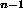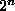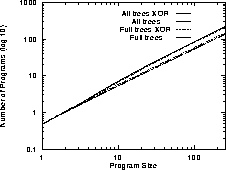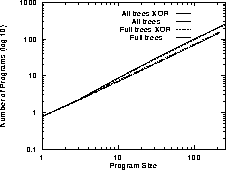Next: Boolean Program Spaces Up: Boolean Functions Fitness Spaces Previous: Introduction

# Boolean Functions

The Boolean functions have often been used as benchmark problems.

The program trees we will consider are composed of n terminals (D0, D1, ... D) which are the Boolean inputs to the program and the Boolean logic functions AND, OR, NAND and NOR [Koza1992]. These are sufficient to construct any Boolean function but we shall also investigate including the exclusive-or function (XOR). Note [Koza1992] required all the functions to have the same arity, this is not required in our approach. The fitness of each tree is given by evaluating it as a logical expression for each of thepossible combinations of Dinputs. Its fitness is the number of fitness cases when its output agrees with that of the target Boolean function.

There aredifferent trees of length l [Koza1992, page 213,] [Alonso and Schott1995]. |F| is four (or five if XOR is included). (Note this formula is simple as each function (internal node) has two arguments). The number of programs rises rapidly (approximately exponentially) with increasing program length l (see Figures 1 and 2). Of course if no bounds are placed on the size or depth of programs then the number of them is unbounded, i.e. the search space is infinite.Figure 1:   Size of 3-Input Boolean Function Search Spaces (note log log scale)Figure 2:   Size of 6-Input Boolean Function Search Spaces (note log log scale)

William B Langdon
Tue Jun 16 15:05:48 BST 1998